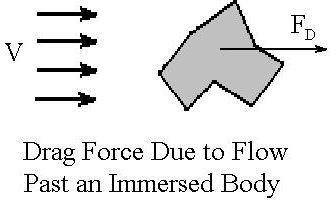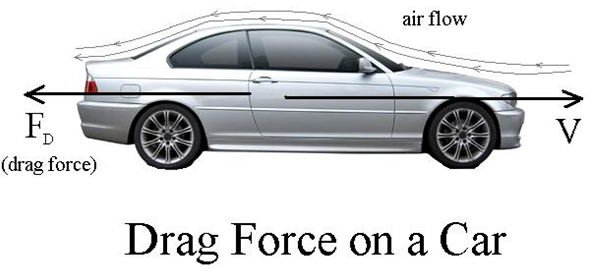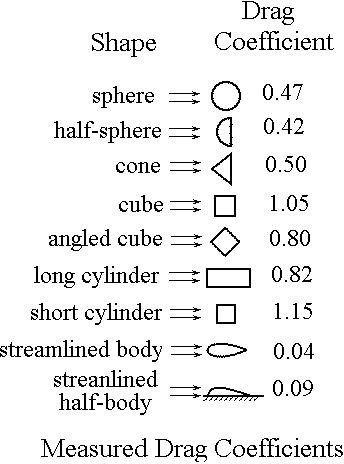# Use of a Drag Coefficient to Calculate Drag Force due to Fluid Flow past an Immersed Solid

## Introduction

When there is fluid flow past a solid object, the resulting air resistance or water resistance causes a drag force. This drag force is of concern for a variety of applications, such as wind force on structures and drag force of air or water on moving vehicles. This article includes the drag force equation, brief discussion of the parameters in the equation, example drag coefficient values and an example drag force calculation.

## The Drag Force EquationThe general equation for the drag force of a fluid flowing past an immersed solid is: FD = CD(1/2)ρV2A

where: FD is the drag force in lb, ρ is the fluid density in slugs/ft3,

A is a reference area as defined for the particular solid in ft2. For many types of immersed objects, thereference area is the frontal area of the object normal to the direction of fluid flow.

CD is the drag coefficient, a dimensionless number, which depends upon the shape of the solid object and perhaps upon the Reynolds Number for the fluid flow. The diagram at the left shows the parameters for drag force due to flow past an immersed body. At the right is a figure showing air flow and drag force due to air resistance for a moving car.

## Drag Coefficient Values

Empirical values for drag coefficient for various shaped objects are given in the diagram at the right and the tablebelow. Note that a value for Reynolds Number is given for some of the shapes. The drag coefficient, in general, depends upon the Reynold’s Number as well as the shape of the object. The effect of Reynolds Number is more important in some cases than others. Reynolds Number for flow past an immersed body is defined as Re = LVρ/μ, where

L is a characteristic dimension for the object in ft

V is the approach velocity of the fuid in ft/sec

ρ is the fluid density in slugs/ft3 and μ is the fluid viscosity in lb-sec/ft2.

• Object — (CD)
• A typical bicycle plus cyclist — (0.9)
• Rough sphere (Re = 1,000,000) — (0.4)
• smooth sphere (Re = 1,000,000) — (0.1)
• Turbulent flow parallel to a flat plate (Re = 1,000,000) — (0.005)
• Laminar flow parallel to a flat plate (Re = 1,000,000) — (0.001)
• Hummer H2 (2003) — (0.57)
• Ford Mustang Fastback (1979) — (0.44)
• Chevrolet Camaro (1995) — (0.338)
• Toyota Prius (2004 – 2009) — (0.26)
• Bullet at subsonic velocity — (0.295)
• Man in upright position — (1.0 – 1.3)
• Flow perpendicular to a long flat plate — (1.98 – 2.0)
• Skier — (1.0 – 1.1)
• Wires and cables — (1.0 – 1.3)
• Empire State Building — (1.3 – 1.5)
• Eiffel Tower — (1.8 – 2.0)

## Example Calculation

Problem: Calculate the drag force due to air resistance on a 3" diameter rough sphere moving through the air at 90 miles per hour, with an air temperature of 50oF and air pressure of 14.7 psi.

Solution: The density of air at the specified conditions can be calculated using the ideal gas law: ρ = (MW)(P/RT) = (29)(14.7/([345.23(50 + 459.67)] = 0.002423 slugs/ft3. (See the article: Use the ideal gas law to find the Density of Air at Different Pressures and Temperatures for more details.)

The velocity needs to be converted to ft/sec: V = (90 mi/hr)(5280 ft/mi)/(3600 sec/hr) = 132 ft/sec.

The frontal area of the sphere is: A = ΠD2/4 = Π(3/12)2/4 = 0.04906 ft2.

The value of the drag coefficient, CD, from the table above is 0.4

Finally, substitute values into the drag force equation: FD = CD(1/2)ρV2A = (0.4)(1/2)(0.002423)(1322)(0.04906) = 0.414 lb